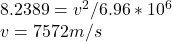## The sputnik one satellite orbiting earth mass equals 5.98×10 to the 24th power kilograms in a circle of radius 6.96×10 to the six power mete

Question

The sputnik one satellite orbiting earth mass equals 5.98×10 to the 24th power kilograms in a circle of radius 6.96×10 to the six power meters what was its orbital velocity

in progress 0
2 weeks 2021-09-02T20:48:24+00:00 1 Answers 0 views 0

7572 m/s

Explanation:

The force between two masses separated by a distance r is given as: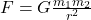Where F is the attractive force between 2 masses, m1 and m2, r is the distance between the centres of the masses and G is the universal gravitation constant, which is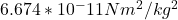The mass of the earth (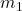) is far greater than the mass of the sputnik (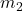). Therefore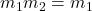. The mass of the sputnik is neglected, therefore: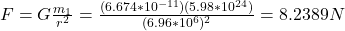But F is actually centripetal acceleration, a = v²/r